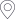﻿ 侯延仁教授学术报告-烟台大学 数学与信息科学学院首页 > 科学研究 > 学术报告 > 正文

## 侯延仁教授学术报告

In this talk, an expandable local and parallel two-grid finite element scheme based on superposition principle for the Stokes equations is proposed and analyzed. Compared with the usual local and parallel finite element schemes, the scheme considered here can be easily implemented in a large parallel computer system, which has a lot of computing cores. Convergence results base on $H^1$ and $L^2$ a priori error estimation of the scheme are obtained, which show that the scheme can produce approximate solution with optimal convergence orders within $|\ln H|^2$ or $|\ln H|$ times two-grid iterations if the coarse mesh size $H$ and the fine mesh size $h$ are properly configured in 2-D or 3-D case, respectively. Some numerical results are presented to support the analysis results.